Courses

# Test: Simple Interest- 1

## 10 Questions MCQ Test General Aptitude for GATE 2020 | Test: Simple Interest- 1

Description
This mock test of Test: Simple Interest- 1 for Quant helps you for every Quant entrance exam. This contains 10 Multiple Choice Questions for Quant Test: Simple Interest- 1 (mcq) to study with solutions a complete question bank. The solved questions answers in this Test: Simple Interest- 1 quiz give you a good mix of easy questions and tough questions. Quant students definitely take this Test: Simple Interest- 1 exercise for a better result in the exam. You can find other Test: Simple Interest- 1 extra questions, long questions & short questions for Quant on EduRev as well by searching above.
QUESTION: 1

### A sum of money at simple interest amounts to Rs. 815 in 3 years and to Rs. 854 in 4 years. The sum is :

Solution:

S.I. for 1 year = Rs. (854 - 815) = Rs. 39.

S.I. for 3 years = Rs.(39 * 3) = Rs. 117.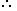Principal = Rs. (815 - 117) = Rs. 698.

QUESTION: 2

### Suresh for 2 years invested Rs. 500 in SBI. He also invested Rs. 300 in ICICI for 4 years. At the end he received Rs. 220 from both banks as simple interest. What must have been rate of interest? (assuming the rate of interest for both banks is same)

Solution: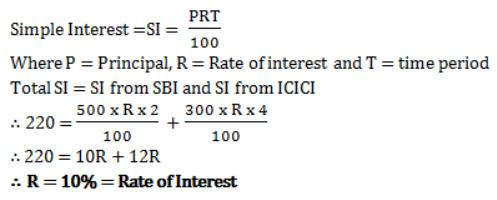QUESTION: 3

### Mr. Thomas invested an amount of Rs. 13,900 divided in two different schemes A and B at the simple interest rate of 14% p.a. and 11% p.a. respectively. If the total amount of simple interest earned in 2 years be Rs. 3508, what was the amount invested in Scheme B?

Solution: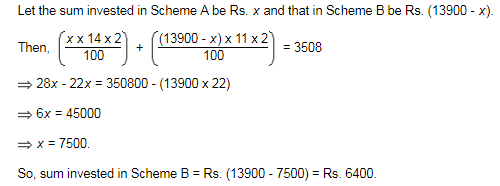QUESTION: 4

A man took loan from a bank at the rate of 8% p.a. simple interest. After 4 years he had to pay Rs. 6200 interest only for the period. The principal amount borrowed by him was:

Solution:

SI=PRT/100
SIx100/RT=P
P=6200*100/32
19375

QUESTION: 5

Arun took a loan of Rs. 1400 with simple interest for as many years as the rate of interest. If he paid Rs.686 as interest at the end of the loan period, what was the rate of interest?

Solution:

Simple Interest (SI) = P N R / 100

P is the Principal loan amount = Rs.1400

N is the number of years of deposit

R is the rate of interest

It is given that the loan period is as many years as the rate of interest.

So, N = R

Interest at the end of the loan period (SI ) = Rs.686

So,

686 = 1400 * R * R /100

R^2 = 686*100 /1400

R^2 = 49

R = 7%

QUESTION: 6

A sum fetched a total simple interest of Rs. 929.20 at the rate of 8 p.c.p.a. in 5 years. What is the sum?

Solution: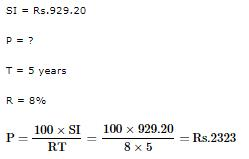QUESTION: 7

Q. How much time will it take for an amount of Rs. 900 to yield Rs. 81 as interest at 4.5% per annum of simple interest?

Solution:

P = Rs.900

SI = Rs.81

T = ?

R = 4.5%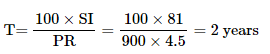QUESTION: 8

What will be the ratio of simple interest earned by certain amount at the same rate of interest for 5 years and that for 15 years?

Solution: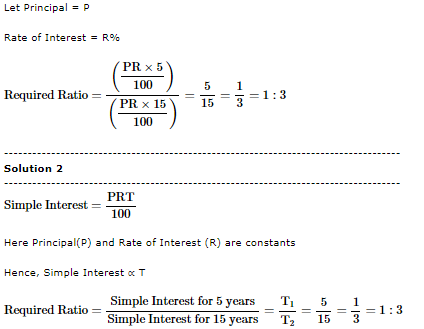QUESTION: 9

A sum of money amounts to Rs.9800 after 5 years and Rs.12005 after 8 years at the same rate of simple interest. The rate of interest per annum is

Solution:

We can get SI of 3 years = 12005 - 9800 = 2205

SI for 5 years = (2205/3)*5 = 3675 [so that we can get principal amount after deducting SI]

Principal = 9800 - 3675 = 6125

So Rate = (100*3675)/(6125*5) = 12%

QUESTION: 10

A certain amount earns simple interest of Rs. 1750 after 7 years. Had the interest been 2% more, how much more interest would it have earned?

Solution:

We need to know the S.I, principal and time to find the rate. Since the principal is not given, so data is inadequate.